# Path Histograms

This demonstrates various options for the path histogram object. In addition to a standard histogram, the path histogram has the ability to interpolate over frames, and can normalize its entries per path instead of per snapshot.

tutorial_path_histogram

# Tutorial: Path Histogram¶

This gives a little overview of the option and behavior of the PathHistogram object. The PathHistogram is used for path density plots and free energy plots. It extends the basic SparseHistogram code by allowing for interpolation between bins, and by allowing one to normalize on a per-trajectory basis.

In :
%matplotlib inline
import matplotlib.pyplot as plt

import openpathsampling as paths
from openpathsampling.analysis import PathHistogram
from openpathsampling.analysis.path_histogram import (
BresenhamInterpolation, BresenhamLikeInterpolation, SubdivideInterpolation
)
from openpathsampling.numerics import HistogramPlotter2D

In :
trajectory = [(0.1, 0.3), (2.1, 3.1), (1.7, 1.4), (1.6, 0.6), (0.1, 1.4), (2.2, 3.3)]
x, y = zip(*trajectory)


Here's our trajectory. The grid happens to correspond with the bins I'll use for the histograms.

In :
plt.grid(True)
plt.plot(x, y, 'o-')

Out:
[<matplotlib.lines.Line2D at 0x11b54b1d0>]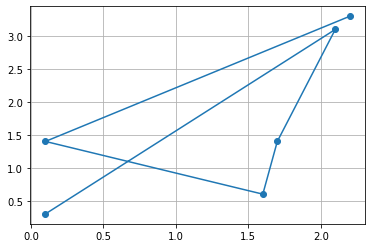The first type of histogram is what you'd get from just histogramming the frames.

In :
hist = PathHistogram(left_bin_edges=(0.0,0.0), bin_widths=(0.5,0.5),
interpolate=False, per_traj=False)

HistogramPlotter2D(hist).plot(normed=False, xlim=(0,2.5), ylim=(0, 3.5),
cmap="Blues", vmin=0, vmax=3)

Out:
<matplotlib.collections.QuadMesh at 0x11b6bdcf8>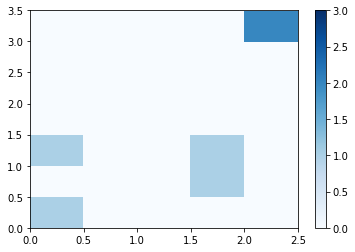The next type of histogram uses that fact that we know this is a trajectory, so we do linear interpolation between the frames. This gives us a count of every time the trajectory enters a given bin. We can use this kind of histogram for free energy plots based on the reweighted path ensemble.

We have several possible interpolation algorithms, so let's show one image for each of them. SubdivideInterpolation is the most exact, but it is also quite slow. The default interpolation is BresenhamLikeInterpolation; this will be used if you just give interpolate=True.

In :
hist = PathHistogram(left_bin_edges=(0.0,0.0), bin_widths=(0.5,0.5),
interpolate=SubdivideInterpolation, per_traj=False)

HistogramPlotter2D(hist).plot(normed=False, xlim=(0,2.5), ylim=(0, 3.5),
cmap="Blues", vmin=0, vmax=3)

Out:
<matplotlib.collections.QuadMesh at 0x11b7964a8>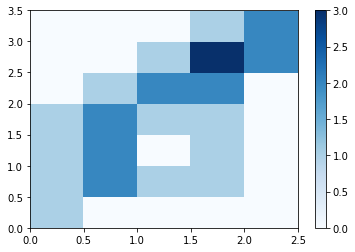In :
hist = PathHistogram(left_bin_edges=(0.0,0.0), bin_widths=(0.5,0.5),
interpolate=BresenhamLikeInterpolation, per_traj=False)

HistogramPlotter2D(hist).plot(normed=False, xlim=(0,2.5), ylim=(0, 3.5),
cmap="Blues", vmin=0, vmax=3)

Out:
<matplotlib.collections.QuadMesh at 0x11b8f4b00>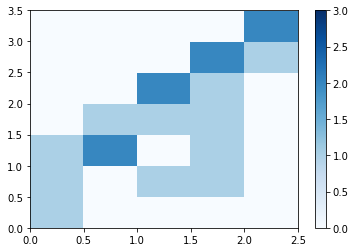In :
hist = PathHistogram(left_bin_edges=(0.0,0.0), bin_widths=(0.5,0.5),
interpolate=BresenhamInterpolation, per_traj=False)

HistogramPlotter2D(hist).plot(normed=False, xlim=(0,2.5), ylim=(0, 3.5),
cmap="Blues", vmin=0, vmax=3)

Out:
<matplotlib.collections.QuadMesh at 0x11b9b49e8>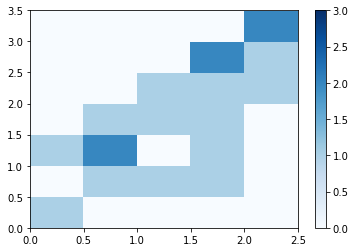The next type of histogram uses the interpolation, but also normalizes so that each trajectory only contributes once per bin. This is what we use for a path density plot.

In :
hist = PathHistogram(left_bin_edges=(0.0,0.0), bin_widths=(0.5,0.5),
interpolate=SubdivideInterpolation, per_traj=True)

HistogramPlotter2D(hist).plot(normed=False, xlim=(0,2.5), ylim=(0, 3.5),
cmap="Blues", vmin=0, vmax=3)

Out:
<matplotlib.collections.QuadMesh at 0x11bad2550>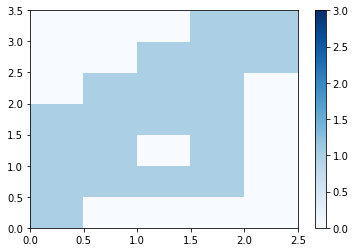Of course, we can normalize to one contribution per path while not interpolating. I don't think this is actually useful.

In :
hist = PathHistogram(left_bin_edges=(0.0,0.0), bin_widths=(0.5,0.5),
interpolate=False, per_traj=True)

HistogramPlotter2D(hist).plot(normed=False, xlim=(0,2.5), ylim=(0, 3.5),
cmap="Blues", vmin=0, vmax=3)

Out:
<matplotlib.collections.QuadMesh at 0x11bc3e7b8>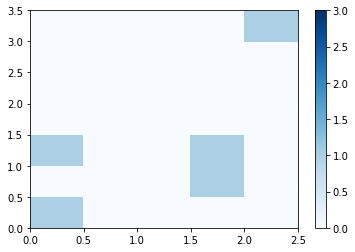Hypothetically, it is possible for a path to cut exactly through a corner. It won't happen in the real world, but we would like our interpolation algorithm to get even the unlikely cases right.

In :
diag = [(0.25, 0.25), (2.25, 2.25)]
diag_x, diag_y = zip(*diag)
plt.grid(True)
ticks = [0.0, 0.5, 1.0, 1.5, 2.0, 2.5, 3.0, 3.5]
plt.xticks(ticks)
plt.yticks(ticks)
plt.xlim(0, 2.5)
plt.ylim(0, 3.5)
plt.plot(diag_x, diag_y, 'o-')

Out:
[<matplotlib.lines.Line2D at 0x11bd84390>]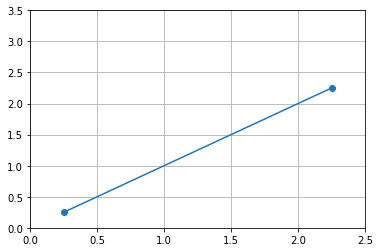In :
hist = PathHistogram(left_bin_edges=(0.0,0.0), bin_widths=(0.5,0.5),
interpolate=SubdivideInterpolation, per_traj=True)

HistogramPlotter2D(hist).plot(normed=False, xlim=(0,2.5), ylim=(0, 3.5),
cmap="Blues", vmin=0, vmax=3)

Out:
<matplotlib.collections.QuadMesh at 0x11bdccb70>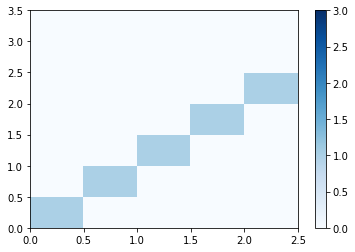How would we make this into an actual path density plot? Add the trajectories on top of each other.

In :
hist = PathHistogram(left_bin_edges=(0.0,0.0), bin_widths=(0.5,0.5),
interpolate=SubdivideInterpolation, per_traj=True)
hist.add_trajectory(diag, weight=2) # each trajectory can be assigned a weight (useful for RPE)

<matplotlib.collections.QuadMesh at 0x11bef5f28>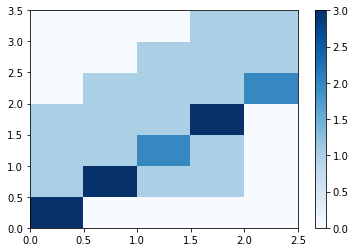The actual PathDensity object also contains information about the collective variables we map this into, and has a convenience function to take a list of regular OPS trajectories and make the whole path histogram out of them.